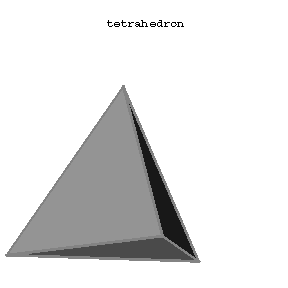# Difference between revisions of "Tetrahedron"

The convex hull of a set of four points, not all in one plane. In particular, a regular tetrahedron is one of the five types of Platonic polyhedra (cf. Platonic solids). A tetrahedron has 4 (triangular) faces, 6 edges and 4 vertices (at each of which 3 edges meet). If $a$ is the length of an edge of the regular tetrahedron, then its volume is

$$\frac{a^3\sqrt2}{12}\approx0.1179a^3.$$

A tetrahedron is a triangular pyramid.Figure: t092510a

A solid tetrahedron is also called a $3$-simplex. The Schläfli symbol of a tetrahedron is $\{3,3\}$.